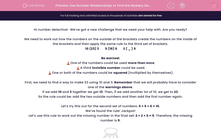# Use Number Relationships to Find the Mystery Number

In this worksheet, students will identify a rule which is used to make a number and apply this rule to find a missing number.This content is premium and exclusive to EdPlace subscribers.Key stage:  KS 2

Curriculum topic:   Verbal Reasoning

Curriculum subtopic:   Number Logic

Difficulty level:#### Worksheet Overview

Hi number detective!  We’ve got a new challenge that we need your help with. Are you ready?

We need to work out how the numbers on the outside of the brackets create the numbers on the inside of the brackets and then apply the same rule to the third set of brackets.

10 (23) 3        5 (16) 6        2 ( _ ) 5

Be warned:One of the numbers could be used more than once.A third invisible number could be used.One or both of the numbers could be squared (multiplied by themselves).

First, we need to find a way to make 23 using 10 and 3. Remember that we will probably have to consider one of the warnings above.

If we add 10 and 3 together we get 13. Then, if we add another lot of 10, we get to 23.

So the rule could be: add the two outside numbers and then add the first number again.

Let’s try this out for the second set of numbers: 5 + 5 + 6 = 16.

We’ve found the rule! Jackpot!

Let’s use this rule to work out the missing number in the final set: 2 + 2 + 5 = 9. Therefore, the missing number is 9.Let’s try another:

5 (28) 3        3 (16) 7         4 ( __ ) 8

First, we need to find a way to make 28 using 5 and 3. Remember that we can use one of the warnings listed above.

Let’s try 5 + 3 = 8 and add the invisible number of 20 to get to 28.

So our rule could be: add the two numbers together and then add the invisible number 20.

Let’s see if this works for the second set of numbers: 3 + 7 = 10 but we need to use a different invisible number of 8 to get to our total.

Bad news, our rule didn’t work so we need to find another.

Let’s try (this means 5 x 5) which gives us 25 and then we need to add 3 to get to 28.

So our rule could be: square the first number and then add the second.

Let’s try this for the second set: is 9 then add 7 gives us 16.

Jackpot! We’ve found the correct rule.

Let’s use this rule to work out the missing number in the final set of numbers: 4² = 16 and then add 8 gives us 24. So the correct answer is 24.Pssstt! Here's a handy hint to help you reach superstar status: Look out for the secret number and don't worry if the first rule you find doesn't work, just stay calm and try another one!

Now it's over to you, to hunt down those missing numbers!

### What is EdPlace?

We're your National Curriculum aligned online education content provider helping each child succeed in English, maths and science from year 1 to GCSE. With an EdPlace account you’ll be able to track and measure progress, helping each child achieve their best. We build confidence and attainment by personalising each child’s learning at a level that suits them.

Get started